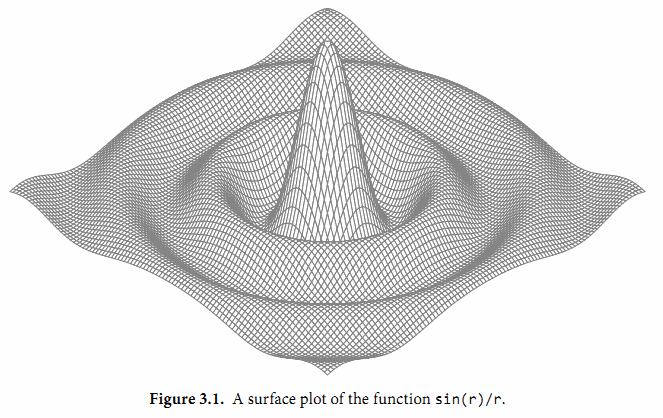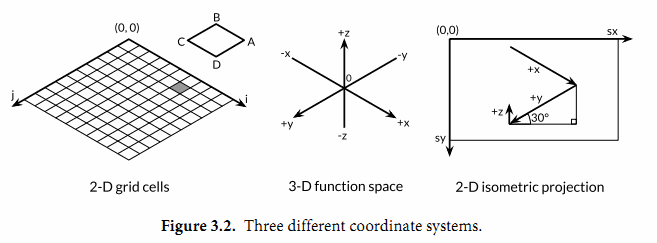## 3.2. 浮点数

Go语言提供了两种精度的浮点数，float32和float64。它们的算术规范由IEEE754浮点数国际标准定义，该浮点数规范被所有现代的CPU支持。

var f float32 = 16777216 // 1 << 24
fmt.Println(f == f+1)    // "true"!


const e = 2.71828 // (approximately)


const Avogadro = 6.02214129e23  // 阿伏伽德罗常数
const Planck   = 6.62606957e-34 // 普朗克常数


for x := 0; x < 8; x++ {
fmt.Printf("x = %d e^x = %8.3f\n", x, math.Exp(float64(x)))
}


x = 0       e^x =    1.000
x = 1       e^x =    2.718
x = 2       e^x =    7.389
x = 3       e^x =   20.086
x = 4       e^x =   54.598
x = 5       e^x =  148.413
x = 6       e^x =  403.429
x = 7       e^x = 1096.633


math包中除了提供大量常用的数学函数外，还提供了IEEE754浮点数标准中定义的特殊值的创建和测试：正无穷大和负无穷大，分别用于表示太大溢出的数字和除零的结果；还有NaN非数，一般用于表示无效的除法操作结果0/0或Sqrt(-1).

var z float64
fmt.Println(z, -z, 1/z, -1/z, z/z) // "0 -0 +Inf -Inf NaN"


nan := math.NaN()
fmt.Println(nan == nan, nan < nan, nan > nan) // "false false false"


func compute() (value float64, ok bool) {
// ...
if failed {
return 0, false
}
return result, true
}gopl.io/ch3/surface

// Surface computes an SVG rendering of a 3-D surface function.
package main

import (
"fmt"
"math"
)

const (
width, height = 600, 320            // canvas size in pixels
cells         = 100                 // number of grid cells
xyrange       = 30.0                // axis ranges (-xyrange..+xyrange)
xyscale       = width / 2 / xyrange // pixels per x or y unit
zscale        = height * 0.4        // pixels per z unit
angle         = math.Pi / 6         // angle of x, y axes (=30°)
)

var sin30, cos30 = math.Sin(angle), math.Cos(angle) // sin(30°), cos(30°)

func main() {
fmt.Printf("<svg xmlns='http://www.w3.org/2000/svg' "+
"style='stroke: grey; fill: white; stroke-width: 0.7' "+
"width='%d' height='%d'>", width, height)
for i := 0; i < cells; i++ {
for j := 0; j < cells; j++ {
ax, ay := corner(i+1, j)
bx, by := corner(i, j)
cx, cy := corner(i, j+1)
dx, dy := corner(i+1, j+1)
fmt.Printf("<polygon points='%g,%g %g,%g %g,%g %g,%g'/>\n",
ax, ay, bx, by, cx, cy, dx, dy)
}
}
fmt.Println("</svg>")
}

func corner(i, j int) (float64, float64) {
// Find point (x,y) at corner of cell (i,j).
x := xyrange * (float64(i)/cells - 0.5)
y := xyrange * (float64(j)/cells - 0.5)

// Compute surface height z.
z := f(x, y)

// Project (x,y,z) isometrically onto 2-D SVG canvas (sx,sy).
sx := width/2 + (x-y)*cos30*xyscale
sy := height/2 + (x+y)*sin30*xyscale - z*zscale
return sx, sy
}

func f(x, y float64) float64 {
r := math.Hypot(x, y) // distance from (0,0)
return math.Sin(r) / r
}(x,y,z)投影到二维的画布中。画布中从远处到右边的点对应较大的x值和较大的y值。并且画布中x和y值越大，则对应的z值越小。x和y的垂直和水平缩放系数来自30度角的正弦和余弦值。z的缩放系数0.4，是一个任意选择的参数。

w.Header().Set("Content-Type", "image/svg+xml")


（这一步在Lissajous例子中不是必须的，因为服务器使用标准的PNG图像格式，可以根据前面的512个字节自动输出对应的头部。）允许客户端通过HTTP请求参数设置高度、宽度和颜色等参数。